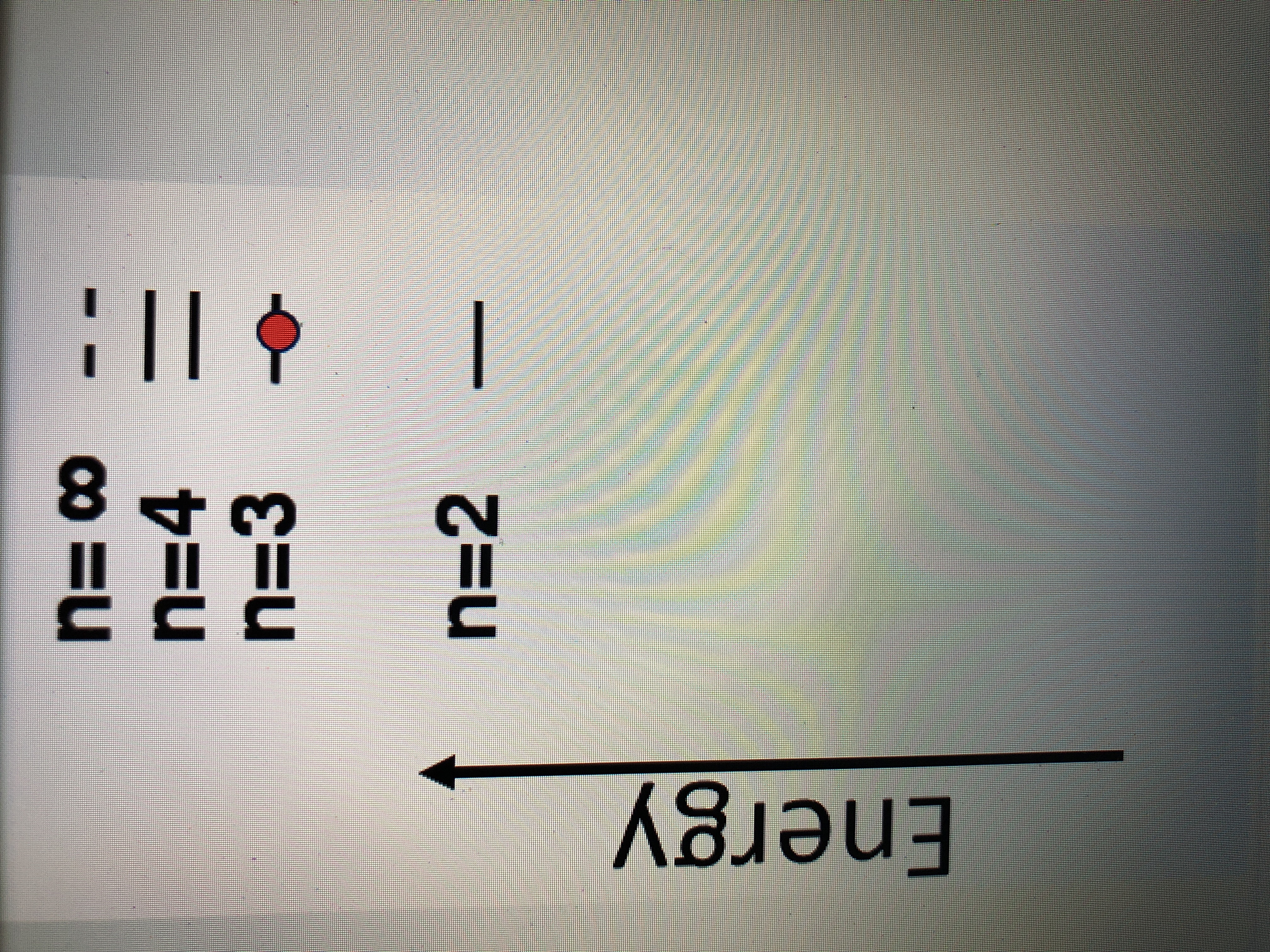# In the energy diagram shown below, the electron is shown (as a red dot) starting in the n = 3 level . If this electron absorbs a photon whose frequency is the longest wavelength that can cause a transition, to which energy level (n) will the electron move? (Select the line that represents the correct energy level in the diagram). N = 1 is at the bottom but I couldn’t fit it in the pic